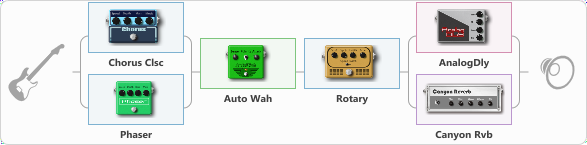# Bass Space

Discussion in 'ToneLib-GFX presets' started by Peter Bass, Mar 17, 2021.

1. Bass Space

Preset name: Bass Space

Effects chain:Effect: "Splitter" (Dynamics / Filter)
{
"A-Bypass" = Off
"A-Pan" = 0
"A-Level" = 55
"B-Bypass" = Off
"B-Pan" = 0
"B-Level" = 55

'A' branch:
{

Effect: "Chorus Clsc" (Modulation / Sfx), active - "yes"
{
"Speed" = 3.5
"Depth" = 58
"Center" = 1.0
"Mode" = Stereo
}
}
'B' branch:
{

Effect: "Phaser" (Modulation / Sfx), active - "yes"
{
"Model" = Boss PH-3
"Speed" = 5.0
"Depth" = 100
"Reso" = 26
"Freq" = 5.0
}
}
}

Effect: "Auto Wah" (Dynamics / Filter), active - "yes"
{
"Sense" = 50
"Polarity" = Up
"Attack" = 42
"Style" = V848
}

Effect: "Rotary" (Modulation / Sfx), active - "yes"
{
"Speed A" = 1.3
"Speed B" = 9.8
"SpeedSw" = A
"Depth" = 50
"Accel" = 5.0
}

Effect: "Splitter" (Dynamics / Filter)
{
"A-Bypass" = Off
"A-Pan" = 0
"A-Level" = 55
"B-Bypass" = Off
"B-Pan" = 0
"B-Level" = 55

'A' branch:
{

Effect: "AnalogDly" (Delay), active - "yes"
{
"Time" = 380
"Feedback" = 34
"Tone" = 65
"Mix" = 65
}
}
'B' branch:
{

Effect: "Canyon Rvb" (Reverberation), active - "yes"
{
"Time" = 6.1
"PreDelay" = 42
"LoDamp" = 0
"HiDamp" = 32
"Mix" = 39
}
}
}

Note: You will need to download and install the ToneLib-GFX software to use the preset.

#### Attached Files:

• ###### Bass_Space.tlgfx
File size:
775 bytes
Views:
2,686
tiltedstudio, SEMENOV and Yurij like this.